### Exponential Reflections

Students determine that the inverse of the exponential function is the natural log function by plotting the inverse of exponential solution points.
•TI-84 Plus CE
•TI-84 Plus C Silver Edition
• TI-84 Plus Silver Edition
• TI-84 Plus### Exponential Growth

Students will find an approximation for the value of the mathematical constant e and to apply it to exponential growth and decay problems.
•TI-84 Plus CE
•TI-84 Plus C Silver Edition
• TI-84 Plus Silver Edition
• TI-84 Plus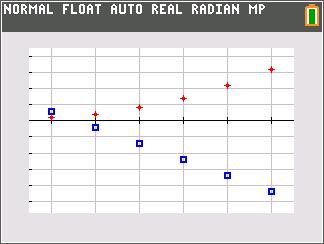### Arithmetic Sequences & Series

Students use formulas to find the differences of the consecutive terms, plot a scatter plot of each sequence, and determine that sequences with common differences (called arithmetic sequences) have scatter plots whose points form a straight line.
•TI-84 Plus CE
•TI-84 Plus C Silver Edition
• TI-84 Plus Silver Edition
• TI-84 Plus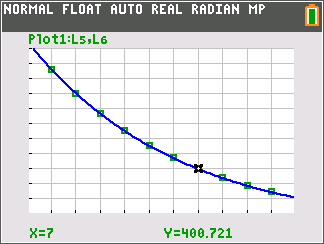### Modeling Data

Students graph data modeling exponential and logarithmic growth and find equations representing the data.
•TI-84 Plus CE
•TI-84 Plus C Silver Edition
• TI-84 Plus Silver Edition
• TI-84 Plus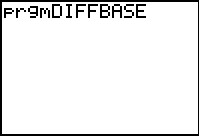### Change Of Base

Discover the change of base rule for logarithms by examining the ratio of two logarithmic functions with different bases.
•TI-84 Plus CE
•TI-84 Plus C Silver Edition
• TI-84 Plus Silver Edition
• TI-84 Plus### Constructing an Ellipse

Students explore two different methods for constructing an ellipse.
• TI-84 Plus Silver Edition
• TI-84 Plus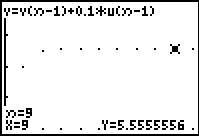### Geometric Sequences & Series

Students find common ratios of geometric sequences on a spreadsheet and create scatter plots of the sequences to see how each curve is related to the value of the common ratio and/or the sign of the first term of the sequence.
•TI-84 Plus CE
•TI-84 Plus C Silver Edition
• TI-84 Plus Silver Edition
• TI-84 Plus### Properties of Logarithms

Students use a combination of algebra and graphing to discover the properties of logarithms.
•TI-84 Plus CE
•TI-84 Plus C Silver Edition
• TI-84 Plus Silver Edition
• TI-84 Plus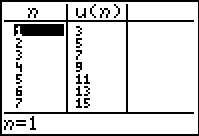### Recursive Sequences

Students use the sequence mode of the graphing calculator to generate recursive sequences and then examine the values.
•TI-84 Plus CE
•TI-84 Plus C Silver Edition
• TI-84 Plus Silver Edition
• TI-84 Plus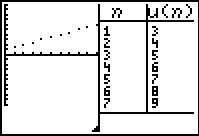### Sequence Investigation

Students use the calculator to create an arithmetic sequence and explore the effect of each variable in the formula of the nth term of an arithmetic sequence.
•TI-84 Plus CE
•TI-84 Plus C Silver Edition
• TI-84 Plus Silver Edition
• TI-84 Plus### Sums of Sequences

Students develop formulas for the sum of arithmetic and geometric sequences and then find the sum of sequences using the formulas developed.
•TI-84 Plus CE
•TI-84 Plus C Silver Edition
• TI-84 Plus Silver Edition
• TI-84 Plus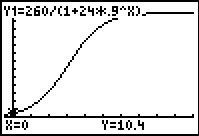••